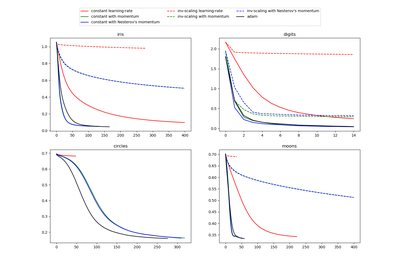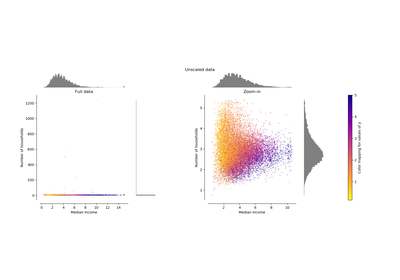# `sklearn.preprocessing`.MinMaxScaler¶

class `sklearn.preprocessing.``MinMaxScaler`(feature_range=(0, 1), copy=True)[source]

Transforms features by scaling each feature to a given range.

This estimator scales and translates each feature individually such that it is in the given range on the training set, i.e. between zero and one.

The transformation is given by:

```X_std = (X - X.min(axis=0)) / (X.max(axis=0) - X.min(axis=0))
X_scaled = X_std * (max - min) + min
```

where min, max = feature_range.

This transformation is often used as an alternative to zero mean, unit variance scaling.

Read more in the User Guide.

Parameters: feature_range : tuple (min, max), default=(0, 1) Desired range of transformed data. copy : boolean, optional, default True Set to False to perform inplace row normalization and avoid a copy (if the input is already a numpy array). min_ : ndarray, shape (n_features,) Per feature adjustment for minimum. scale_ : ndarray, shape (n_features,) Per feature relative scaling of the data. New in version 0.17: scale_ attribute. data_min_ : ndarray, shape (n_features,) Per feature minimum seen in the data New in version 0.17: data_min_ data_max_ : ndarray, shape (n_features,) Per feature maximum seen in the data New in version 0.17: data_max_ data_range_ : ndarray, shape (n_features,) Per feature range `(data_max_ - data_min_)` seen in the data New in version 0.17: data_range_

`minmax_scale`
Equivalent function without the estimator API.

Notes

For a comparison of the different scalers, transformers, and normalizers, see examples/preprocessing/plot_all_scaling.py.

Examples

```>>> from sklearn.preprocessing import MinMaxScaler
>>>
>>> data = [[-1, 2], [-0.5, 6], [0, 10], [1, 18]]
>>> scaler = MinMaxScaler()
>>> print(scaler.fit(data))
MinMaxScaler(copy=True, feature_range=(0, 1))
>>> print(scaler.data_max_)
[  1.  18.]
>>> print(scaler.transform(data))
[[ 0.    0.  ]
[ 0.25  0.25]
[ 0.5   0.5 ]
[ 1.    1.  ]]
>>> print(scaler.transform([[2, 2]]))
[[ 1.5  0. ]]
```

Methods

 `fit`(X[, y]) Compute the minimum and maximum to be used for later scaling. `fit_transform`(X[, y]) Fit to data, then transform it. `get_params`([deep]) Get parameters for this estimator. `inverse_transform`(X) Undo the scaling of X according to feature_range. `partial_fit`(X[, y]) Online computation of min and max on X for later scaling. `set_params`(**params) Set the parameters of this estimator. `transform`(X) Scaling features of X according to feature_range.
`__init__`(feature_range=(0, 1), copy=True)[source]
`fit`(X, y=None)[source]

Compute the minimum and maximum to be used for later scaling.

Parameters: X : array-like, shape [n_samples, n_features] The data used to compute the per-feature minimum and maximum used for later scaling along the features axis.
`fit_transform`(X, y=None, **fit_params)[source]

Fit to data, then transform it.

Fits transformer to X and y with optional parameters fit_params and returns a transformed version of X.

Parameters: X : numpy array of shape [n_samples, n_features] Training set. y : numpy array of shape [n_samples] Target values. X_new : numpy array of shape [n_samples, n_features_new] Transformed array.
`get_params`(deep=True)[source]

Get parameters for this estimator.

Parameters: deep : boolean, optional If True, will return the parameters for this estimator and contained subobjects that are estimators. params : mapping of string to any Parameter names mapped to their values.
`inverse_transform`(X)[source]

Undo the scaling of X according to feature_range.

Parameters: X : array-like, shape [n_samples, n_features] Input data that will be transformed. It cannot be sparse.
`partial_fit`(X, y=None)[source]

Online computation of min and max on X for later scaling. All of X is processed as a single batch. This is intended for cases when fit is not feasible due to very large number of n_samples or because X is read from a continuous stream.

Parameters: X : array-like, shape [n_samples, n_features] The data used to compute the mean and standard deviation used for later scaling along the features axis. y : Passthrough for `Pipeline` compatibility.
`set_params`(**params)[source]

Set the parameters of this estimator.

The method works on simple estimators as well as on nested objects (such as pipelines). The latter have parameters of the form `<component>__<parameter>` so that it’s possible to update each component of a nested object.

Returns: self :
`transform`(X)[source]

Scaling features of X according to feature_range.

Parameters: X : array-like, shape [n_samples, n_features] Input data that will be transformed.

## Examples using `sklearn.preprocessing.MinMaxScaler`¶Compare Stochastic learning strategies for MLPClassifierCompare the effect of different scalers on data with outliers